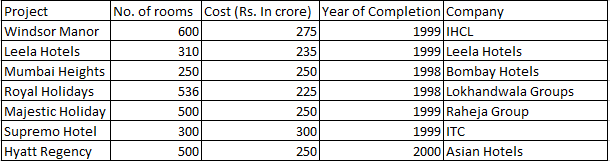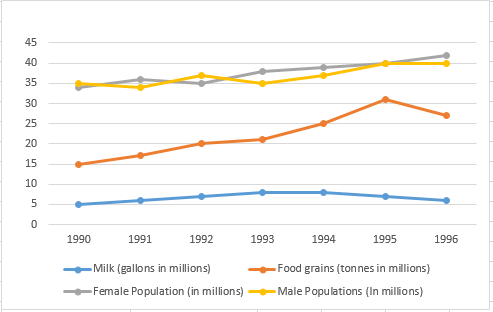# CAT 1997

Instructions

The following table gives the number of rooms in different hotels in Mumbai and the cost of building each one of them. Note that all the projects started in 1997Note: All projects start in 1997.

Question 1

# Which of the following had the least cost per room?Question 2

# Which of the following has the maximum number of rooms per crore of rupees?Question 3

# Assume that the cost of the project is incurred in the year of completion; interest is charged at the rate of 10% per annum. What is the cost incurred for projects completed in 1998?Question 4

# Assume that the cost of the project is incurred in the year of completion; interest is charged at the rate of 10% per annum. What is the cost incurred for projects completed in 1999?Question 5

# What is the approximate cost incurred for projects completed by 2000? (in crores)Instructions

The graph given below shows the quantity of milk and food grains consumed annually along with female and male population (in millions). Use the data to answer the questions that follow.Question 6

# When was the percapita consumption of milk the least?Question 7

# When was the per capita production of foodgrains most?Question 8

# In which year was the difference between the percentage increase in the production of food grains and milk maximum?Question 9

# If milk contains 320 calories and foodgrains contain 160 calories, in which year was the per capita consumption of calories highest?Question 10

# If one gallon milk contains 120 g of a particular nutrient and one tonne of foodgrains contains 80 g of the same nutrient, in which year was the availability of this nutrient maximum?OR